# Least squares fitting (linear/nonlinear)

Linear and nonlinear least squares fitting is one of the most frequently encountered numerical problems. ALGLIB package includes several highly optimized least squares fitting algorithms available in several programming languages, including:

• , a high performance C++ library with great portability across hardware and software platforms
• , a highly optimized C# library with two alternative backends: a pure C# implementation (100% managed code) and a high-performance native implementation (Windows, Linux) with same C# interface

Following algorithms are implemented (see below for more detailed discussion):

• 1-dimensional fitting algorithms, including polynomial fitting, penalized cubic spline fitting, rational fitting
• linear least squares fitting, with additional options like weights, error estimates, linear constraints
• nonlinear least squares fitting with Levenberg-Marquardt algorithm (box and general linear constraints; optional numerical differentiation; verification of user-provided gradient)

# Least squares fitting

## Linear least squares

Most fitting algorithms implemented in ALGLIB are build on top of the linear least squares solver:

• Polynomial curve fitting (including linear fitting)
• Rational curve fitting using Floater-Hormann basis
• Spline curve fitting using penalized regression splines
• And, finally, linear least squares fitting itself

First three methods are important special cases of the 1-dimensional curve fitting. Last method can be used for 1-dimensional or multidimensional fitting.

## Nonlinear least squares

ALGLIB package supports nonlinear fitting by user-defined functions using Levenberg-Marquardt optimizer. Interface described below is just a convenience wrapper around underlying optimizer.

## Choosing fitting algorithm

If you don't know what method to choose or just want to explore all features of the ALGLIB package, you can use our Guide to Fitting Algorithms. If you want:

• to fit smooth function with high precision, then you can try polynomial fitting. For sufficiently smooth function it is possible to reduce relative error up to 10 -14. For example, you can build polynomial model of some transcendent function. However, polynomial fitting is not good if you want to model noisy, oscillating or fast-varying functions. High degree polynomials are prone to different instabilities (numerical errors, Runge's phenomenon). They are not good at fitting non-uniformly distributed (with large gaps) data.
• to fit smooth function with moderate precision and guaranteed convergence and stability, then you can use rational fitting by Floater-Hormann functions. Floater-Hormann basis is well conditioned and is not susceptible to the Runge phenomenon.
• to fit curve to experimental data containing noise, then the best choice is to use penalized regression spline. It is easy to use and to tune, easily copes with large gaps in the data (empty areas where function values are unknown). Smoothing degree can vary in very wide range - from almost 100% damping of all nonlinearities (result is a straight line) to almost 100% absence of smoothing.
• to solve multidimensional problem, then you can use general linear or nonlinear least squares solver. These solvers can fit general form functions represented by basis matrix (LLS) or by callback which calculates function value at given point (NLS).

# Polynomial curve fitting

## Polynomial curve fitting using barycentric representation

You can make polynomial fit with polynomialfit (unconstrained unweighted fitting) and polynomialfitwc (constrained weighted fitting) functions. Second function supports arbitrary number of constrains on function value - f(xc)=yc - or its derivative - df(xc)/dx=yc. Time needed to solve problem is O(N·M 2) (where N is the number of points, M is the basis size).

Note #1
Excessive constraints can be inconsistent, especially when you fit data by low degree polynomial. Read comments on polynomialfitwc for more information on this subject.

Both functions use Chebyshev basis with automatic scaling/translation internally, which allows to reduce condition number. However, they return fitting result in the barycentric form, which is used by ALGLIB to store polynomial and rational functions.

## Conversion to power basis

ALGLIB package uses barycentric representation for polynomial operations because it is more stable than the power basis representation. Coefficients of barycentric model are stored in the barycentricinterpolant object. There are two subpackages - polint and ratint - which provide functions for operations with this object (evaluation, differentiation, etc.). More information on this subject can be obtained in the User Guide on polynomial interpolation.

However, sometimes users need polynomial coefficients, not some black box which can be used to evaluate polynomial at any point. You can use two functions to do so:

• polynomialbar2pow - to convert from barycentric representation to power basis
• polynomialbar2cheb - to convert from barycentric representation to Chebyshev basis

## Examples

Examples below describe polynomial fitting. If you want to know about polynomial interpolation in the ALGLIB, we can recommend you to read polint subpackage description from ALGLIB Reference Manual or to read ALGLIB User Guide on polynomial interpolation.

• lsfit_d_pol example demonstrates unconstrained polynomial fitting
• lsfit_d_polc example demonstrates constrained polynomial fitting

# Rational curve fitting

## Rational curve fitting using Floater-Hormann basis

ALGLIB package includes two functions for rational curve fitting using Floater-Hormann basis: barycentricfitfloaterhormann, which solves unconstrained unweighted problems, and barycentricfitfloaterhormannwc, which solves problem with individual weighs and constraints. Latter one supports arbitrary number of constraints on function value or first derivative: f(xc)=yc or df(xc)/dx=yc. As result, barycentricinterpolant object is returned. You can use functions provided by ratint subpackage to work with barycentric interpolant (evaluate, differentiate, etc.). More information on this subject can be obtained in the User Guide on rational interpolation.

Note #2

Both functions use Floater-Hormann basis built on equidistant grid with M nodes. They search for optimal D in [0,9] ("optimal" means "least weighted RMS error"). Because such fitting task is linear, linear solver with O(N·M 2) complexity is used (here N is a number of points, M is a basis size).

It is important to note that reduced set of rational functions is used for fitting - set of Floater-Hormann rational functions, which have no poles on the real axis. From the one side, Floater-Hormann fitting is more stable than fitting by arbitrary rational functions. From the other side, Floater-Hormann functions have less degrees of freedom than general rational function. You may achieve error as small as 10 -3...10 -5 using only moderate number of points. But interpolation/fitting error have geometric, not exponential convergence, thus Floater-Hormann function are not well suited for tasks where extra high precision with limited number of points is needed.

# Cubic spline curve fitting

## Overview

ALGLIB package supports three types of spline fits:

• penalized regression spline fitting
• unpenalized regression spline fitting
• Hermite fitting

Last two algorithms are inferior to the first one and are not described here. They are still supported by ALGLIB, but because of backward compatibility only.

## Penalized regression spline

Penalized regression spline is a 1-dimensional curve fitting algorithm which is suited for noisy fitting problems, underdetermined problems, and problems which need adaptive control over smoothing. It is cubic spline with continuous second derivative, with M uniformly distributed nodes, whose coefficients are obtained as minimizer of sum of LS (approximation error) and P (penalty function which suppresses nonlinearity):You can built penalized regression spline with two functions:

• spline1dfitpenalized, which solves unweighted problem
• spline1dfitpenalizedw, which solves weighted problem

Both functions reduce minimization of LS+P to the linear least squares problem with O(N·M 2+M 3) time complexity. As result, they return spline1dinterpolant object which contains cubic spline. ALGLIB package offers a lot of functions which operate with this object. They are described in documentation on spline1d subpackage. Some additional information can be found in the User Guide on cubic splines.

## Benefits

Key benefits of penalized regression spline are:

• adaptive control over nonlinearity and noise suppression. You can control smoothing by varying ρ.
• ability to solve both overdetermined and underdetermined problems. Unlike with other fitting types (polynomial, rational), you can increase number of basis functions without risk of overfitting. You can make number of degrees of freedom M several times larger than sample size N - and get smooth fit. This is achieved by using penalty function which penalizes too sharp fluctuations in the spline and improves condition number of linear systems being solved. Such result is almost impossible to achieve with polynomial fitting.
• simple tuning - only two parameters to tune, M and ρ, with no cross-dependencies between them (see below)

Penalized regression spline is quite different from other fitting algorithms. It is one of the best one dimensional fitting algorithms. If you need stable and easy to tune fitting algo, we recommend you to choose penalized splines.

## Tuning

As many other algorithms, penalized spline needs some simple tuning:

1. choose number of degrees of freedom M. Nodes count must be large enough to provide desired flexibility, and small enough for good performance. However, there is no such thing as too large M - penalty function will prevent spline from being too flexible. You can start from M=100 and increase it by 100 each time, until you decide that it is flexible enough. If performance is not a problem, you can try M=3·N - and it will work.
2. choose regularization coefficient ρ. Larger values of ρ correspond to larger degrees of smoothing. Very large value of ρ will result in straight line fit. This coefficient is automatically scaled so that its values are in the [-15,+15] range. Most practical applications, however, use values from [-2,+6] range. You can start from ρ=0.0.

Thus, algorithm tuning includes two independent stages. Parameters are tuned separately, and you can set M in the "set and forget" fashion, concentrating on tuning of the ρ parameter.

## Example

Consider application of penalized regression spline to the smoothing of noisy data. We will try to fit f, given by its values on N equidistant nodes, by spline with M degrees of freedom and different values of penalty coefficient ρ.f is composed from three terms: the first one is low-frequency part, the second one is medium-frequency noise, and the third one is high frequency noise. On the first of two charts below you can see low-frequency part (blue curve) and noisy function we pass to the smoother (red curve). Two harmonics are clearly seen in the noise - one with frequency equal to 10, and another with frequency equal to 100.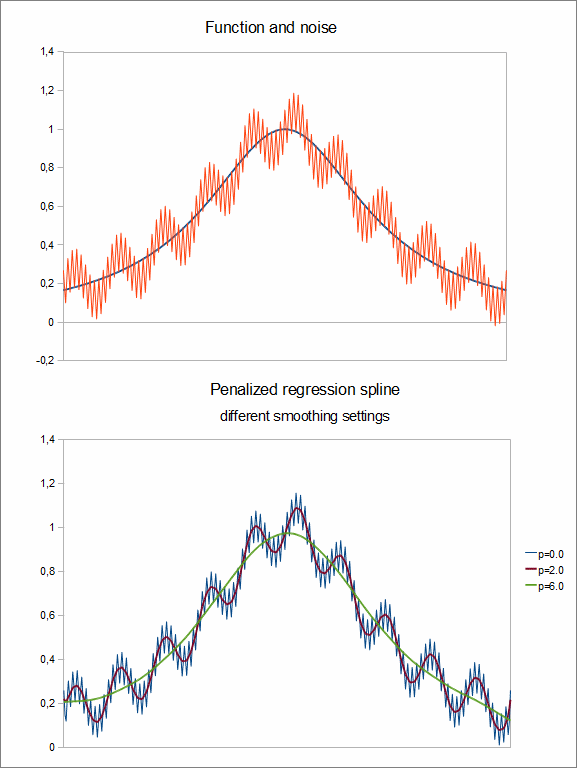Second chart shows us three splines corresponding to different values of ρ. Blue curve was generated using very low value of ρ (although it is not the lowest value possible - ρ can be negative). Dark red line was generated with moderate amount of smoothing, which damped high frequency components, but left medium frequency components almost unchanged. And finally, green curve was generated with high amount of smoothing, which fully suppressed noise in the data.

Note #3
Take into the account that number of degrees of freedom M was significantly larger than number of points N, but we see no signs of overfitting.

We do not provide source code for example above because it does not fit into our automated multilingual example generation framework. However, ALGLIB reference manual includes other spline-related examples with source code:

• lsfit_d_spline example, which shows how to use penalized regression spline for unconstrained fits

# Linear least squares fitting

## Unconstrained problems

ALGLIB package contains two functions for solution of the unconstrained linear least squares problems:

• lsfitlinear, which solves unweighted problems
• lsfitlinearw, which solves weighted problems

Linear least squares fitting can be used if function being fitted is represented as linear combination of basis functions. Basis functions themselves can be nonlinear with respect to x.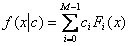For example, fPOL  (see below), demonstrates that polynomial is actually linear function with respect to its coefficients c. Another function, fSIN , demonstrates usage of sines as basis functions .The solution of the least squares problem is c - vector of coefficients which minimizes fitting error E(x). Below you can find two formulae for E(x) - one for weighted problem, another for unweighted one: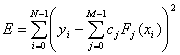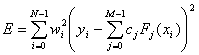Note #4
Note that weights, if there are any, are squared.

Minimizer of such function can be found by solution of linear system, which allows us to use linear solver which has fixed O(N·M 2) time complexity. We use direct solver, so you don't have to wait some unknown amount of time for convergence of iterative algorithm. Another benefit, arising from problem linearity, is that you don't have to repeatedly calculate values of basis functions. Just calculate them once in all sample points and pass matrix of values to the algorithm.

Note #5
We use SVD-based solver, which allows us to solve even degenerate problems with linearly dependent systems of basis functions.

Note #6
Most fitting algorithm described on this page are actually wrappers around linear solver described here.

## Problems with linear equality constraints

If you have LLS problem with linear equality constraints on coefficient vector c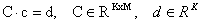you can use:

• lsfitlinearc, to solve unweighted linearly constrained problem
• lsfitlinearwc, to solve weighted linearly constrained problem

As in unconstrained case, problem reduces to the solution of the linear system.

Note #7
Constraints can be inconsistent, i.e. there may not exists such c which satisfies all of them simultaneously. In this case, corresponding error code will be returned.

## Examples

This section contains links to examples of linear least squares fitting:

• lsfit_d_lin example, which show how to do unconstrained LLS fits
• lsfit_d_linc example, which show how to do constrained LLS fits

# Fast fitting with RBF models

RBF models allow to approximate scalar or vector functions in 2D or 3D space. This method has no limitations on location of points, which can be sampled irregularly or even be non-distinct. It makes RBF models interesting alternative to another algorithms, like 2D/3D approximating splines. ALGLIB package implements efficient RBF fitting algorithm with O(N·logN) working time (rbf unit). This algorithm is studied in more details in the article on ALGLIB implementation of RBFs, which we recommend to anyone who have to solve fitting problems in 2D/3D.

# Nonlinear least squares fitting

## Overview

ALGLIB package supports nonlinear curve fitting using Levenberg-Marquardt method. Nonlinear fitting is supported by lsfit subpackage which provides several important features:

• unconstrained and bound constrained fitting
• scaling of the variables (in order to handle problems where variables differ in magnitudes)

Nonlinear fitting is quite different from linear one: 1) linear problems have fixed time complexity, whereas solution of nonlinear problem is an iterative process, whose convergence speed is problem-dependent, and 2) nonlinear methods generally have more tunable parameters than linear ones. Linear problems are solved in one step - we pass data to the solver and get our result. Nonlinear fitting includes several steps:

1. first, we create solver object using one of the constructor functions
2. then we tune solver, set stopping conditions and/or other parameters
3. then we start solution by calling lsfitfit function
4. finally, after return from lsfitfit, we read solution result by calling lsfitresults

## Choosing operating modes

ALGLIB users can choose between three operating modes of nonlinear solver which differ in what information about function being fitted they need:

• F-mode, when only function value is needed. User don't have to calculate analytic gradient/Jacobian - it will be done automatically using combination of numerical differentiation (for precision) and secant updates (for better speed). It is the slowest, but the simplest operating mode provided by ALGLIB nonlinear solver.
• FG-mode; in this mode user should implement calculation of both function and its analytic gradient. This mode is faster than F-mode and can find solution with better accuracy.
• FGH-mode, when user should provide function value, analytic gradient, analytic Hessian. This mode can be beneficial when you have high level of noise in your data and cheap Hessian.

Any of the modes mentioned above can be used to solve unweighted or weighted problems. Letters in the mode name are appended to the constructor function name; if you use weighted version, W is appended too. So we have 6 versions of constructor functions:

• lsfitcreatef - nonlinear curve fitting using function value f(x|c) only
• lsfitcreatewf - nonlinear curve fitting using function value f(x|c) only, weighted setting
• lsfitcreatefg - nonlinear curve fitting using function value f(x|c) and gradient with respect to c
• lsfitcreatewfg - nonlinear curve fitting using function value f(x|c) and gradient with respect to c, weighted setting
• lsfitcreatefgh - nonlinear curve fitting using function value f(x|c), gradient and Hessian with respect to c
• lsfitcreatewfgh - nonlinear curve fitting using function value f(x|c), gradient and Hessian with respect to c, weighted setting

What operating mode to choose? For a quick start we recommend to choose F-mode, because it is the simplest of all nonlinear fitting modes provided by ALGLIB. You just calculate function value at given point x with respect to the vector of tunable parameters c, and ALGLIB package solves all numerical differentiation issues. However, as we told above, gradient-free nonlinear fitting is easy to use, but is not efficient. Furthermore, numerical differentiation doesn't allow us to find solution with accuracy significantly higher than numerical differentiation step. Thus if you need high performance or high accuracy, you should implement calculation of analytic gradient and switch to FG-mode.

## Good fit vs .bad fit

It is well known that Levenberg-Marquardt method converges quadratically when all points are close to the best-fit curve ("good fit"). However, on a "bad fit" problems convergence becomes linear. If you (a) need very good performance on a "bad fit" problems and (b) have cheap Hessian, you can try using FGH-mode. It requires less iterations, but iteration cost is somewhat higher, so it is hard to tell whether it will be beneficial or not. However, in some situations it is worth a try.

## Choosing stopping criteria

ALGLIB package offers three types of stopping criteria:

• stop after sufficiently small step
• stop after sufficiently small function change
• stop after specified number of iterations

You may set one or several criteria by calling lsfitsetcond function. After algorithm is done, you can analyze completion code and determine why it stopped.

We recommend you to use first criterion (sufficiently small step). Step size is problem dependent, but we recommend to use some small value, significantly smaller than desired accuracy. Sometimes, in the hard places, algorithm can make very small step. And if length of last step was 0.001, it does not mean that distance to the solution has same magnitude. For example, it can mean that our quadratic model is too old and needs recalculation. Or that we move through valley with hard turns.

Note #8
Additionally you may limit number of algorithm iterations - if you want to guarantee that algorithm won't work for too long. For example, such criterion is useful in some embedded/real-time applications where you need something right now - or nothing at all.

Note #9
"sufficiently small gradient" criterion is inaccessible to user because it is hard to tell what value of "gradient of weighted sum of squared residuals" is sufficiently small. Stepsize or function change criteria are more intuitive.

## Scaling variables

Before you start to use optimizer, we recommend you to set scale of the variables with lsfitsetscale function. Scaling is essential for correct work of the stopping criteria (and sometimes for convergence of optimizer). You can do without scaling if your problem is well scaled. However, if some variables are up to 100 times different in magnitude, we recommend you to tell solver about their scale. And we strongly recommend to set scaling in case of larger difference in magnitudes.

We recommend you to read separate article on variable scaling, which is worth reading unless you solve some simple toy problem.

ALGLIB package supports fitting with boundary constraints, i.e. constraints of the form l≤c≤u. Boundary constraints can be set with lsfitsetbc function. These constraints are handled very efficiently - computational overhead for having N constraints is just O(N) additional operations per function evaluation. Finally, these constraints are always exactly satisfied. We won't calculate function at points outside of the interval given by [l,u]. Optimization result will be inside [l,u] or exactly at its boundary.

• set maximum step length by calling lsfitsetstpmax function. Upper limit on step size is useful when fitting fast growing functions (e.g., sum if exponentials), when too large step may lead to overflow.
• request reports after each iteration by calling lsfitsetxrep function.

## Increasing performance

Nonlinear least squares solver described here is actually a convenience wrapper around Levenberg-Marquardt optimizer. Working with specialized interface is more convenient that using underlying optimization algorithm directly. From the other side, convenience interface is somewhat slower than original algorithm because of additional level of abstraction it provides. When Levenberg-Marquardt algorithm makes one call of user-defined function, convenience wrapper makes N calls (N is a number of points), each of them being accompanied with complex movement of data between internal structures. It is especially important for small-scale problems (1-3 parameters to fit) with very cheap functions/gradients - in such cases performance may be up to several times lower.

What to choose - performance or convenience? If you are new to ALGLIB and want to quickly get some code which works, we recommend you to use nonlinear least squares solver described here. You will save some development time and you will be able to qiuckly build working prototype.

However, if you need high performance, we recommend you to work directly with underlying optimizer. Or alternatively you can start from lsfit subpackage (specialized interface) and, after getting familiar with ALGLIB, switch to minlm subpackage (underlying optimizer).

## Examples

This section contains links to examples of nonlinear least squares fitting:

• lsfit_d_nlf example, which demonstrates gradient-free fitting (F-mode)
• lsfit_d_nlfg example, which demonstrates nonlinear fitting using analytic gradient (FG-mode)
• lsfit_d_nlfgh example, which shows how to fit using analytic gradient and Hessian (FGH-mode)
• lsfit_d_nlfb example, which shows how to do bound constrained fitting
• lsfit_d_nlscale example, which demonstrates badly scaled fitting problem (variables have different magnitudes)

ALGLIB Project offers you two editions of ALGLIB:

ALGLIB Free Edition:delivered for freeoffers full set of numerical functionalityextensive algorithmic optimizationsno low level optimizationsnon-commercial license

ALGLIB Commercial Edition:flexible pricingoffers full set of numerical functionalityextensive algorithmic optimizationshigh performance (SMP, SIMD)commercial license with support plan

## ALGLIB 3.16.0 for C++C++ library.
Delivered with sources.
Monolithic design.
Extreme portability.
Editions:   FREE   COMMERCIAL

## ALGLIB 3.16.0 for C#C# library with native kernels.
Delivered with sources.
VB.NET and IronPython wrappers.
Extreme portability.
Editions:   FREE   COMMERCIAL

## ALGLIB 3.16.0 for DelphiDelphi wrapper around C core.
Delivered as precompiled binary.
Compatible with FreePascal.
Editions:   FREE   COMMERCIAL

## ALGLIB 3.16.0 for CPythonCPython wrapper around C core.
Delivered as precompiled binary.
Editions:   FREE   COMMERCIAL